Next: Generalized Force Balance Equations Up: Background Previous: Background

## Parametric Snake Model

In the original snake formulation of Kass et al. , the best snake position was defined as the solution of a variational problem requiring the minimization of the sum of internal and external energies integrated along the length of the snake. The corresponding Euler equations, which give the necessary conditions for this minimizer, comprise a force balance equation. By introducing a temporal parameter t, the force balance equation can be made dynamic. When the dynamic equation reaches its steady state, a solution to the static problem is found. We now give a brief summary of these steps.

A snake is a curve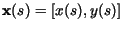,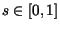, that moves through the spatial domain of an image to minimize the energy functional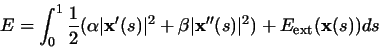(1)

where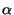and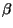are weighting parameters that control the snake's tension and rigidity, respectively.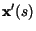and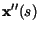denote the first and second derivatives of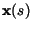with respect to s. The external energy function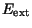is derived from the image so that it takes on its smaller values at the features of interest, such as boundaries.

Given a gray-level image I(x,y) (viewed as a function of continuous position variables (x,y)), typical external energies designed to lead an active contour toward step edges are :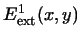=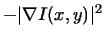(2)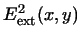=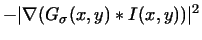(3)

where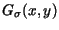is a two-dimensional Gaussian function with standard deviation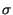and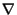is the gradient operator. If the image is a line drawing (black on white), then appropriate external energies include :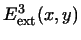= I(x,y) (4)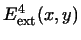=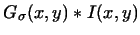(5)

It is easy to see from these definitions that larger's will cause the boundaries to become blurry. Such large's are often necessary, however, in order to make the effect of the boundary felt'' at some distance from the boundary -- i.e., to increase the capture range of the active contour.

A snake that minimizes E must satisfy the Euler equation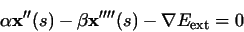(6)

This can be viewed as a force balance equation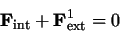where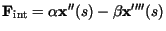and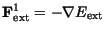. The internal force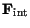discourages stretching and bending while the external force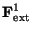pulls the snake towards the desired image contour.

To find a solution to (6), the snake is made dynamic by treating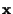as function of time t as well as s -- i.e.,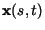. Then, the partial derivative ofwith respect to t is then set equal to the left hand side of (6) as follows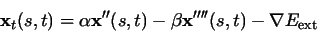(7)

When the solutionstabilizes, the term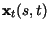vanishes and we achieve a solution of (6). This dynamic equation can also be viewed as a gradient descent algorithm  designed to solve (1). A solution to (7) can be found by discretizing the equation and solving the discrete system iteratively (cf. ).Next: Generalized Force Balance Equations Up: Background Previous: Background
Chenyang Xu
1999-11-06